# Chapter 14

30 de Dec de 2014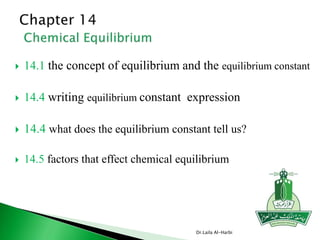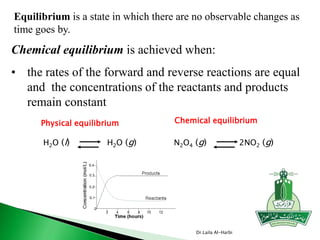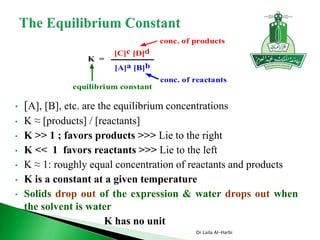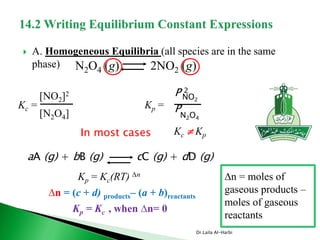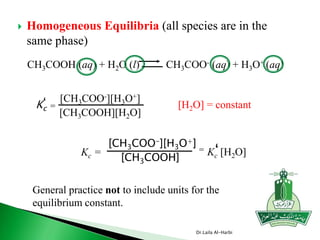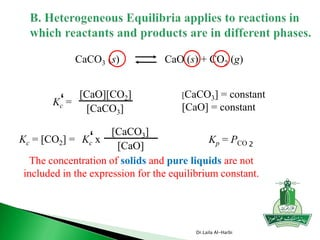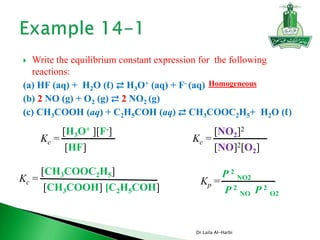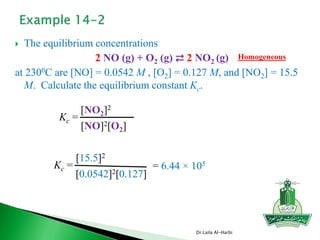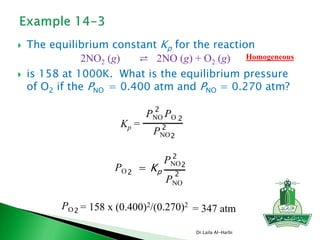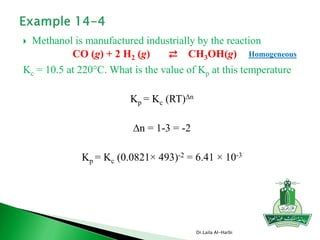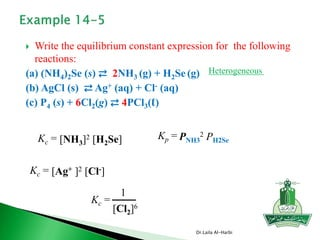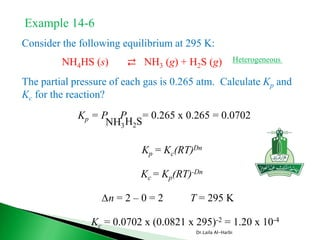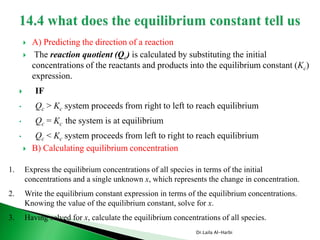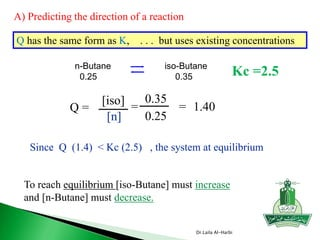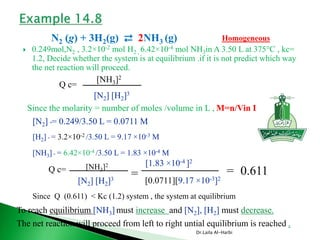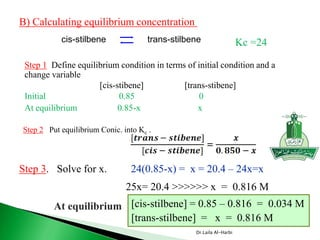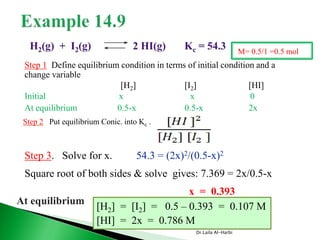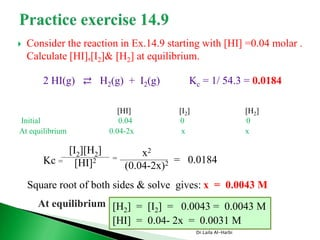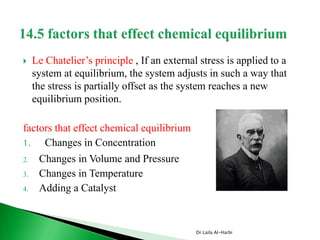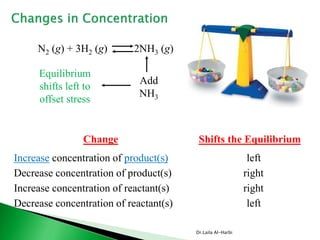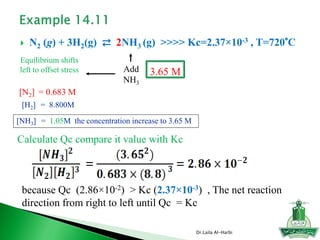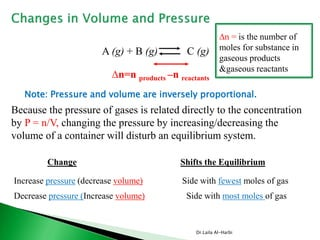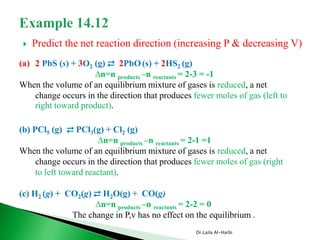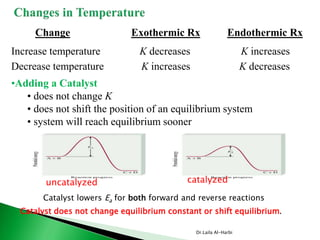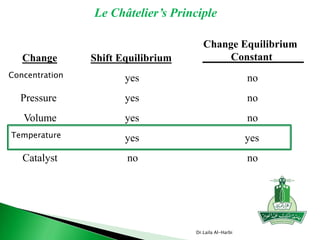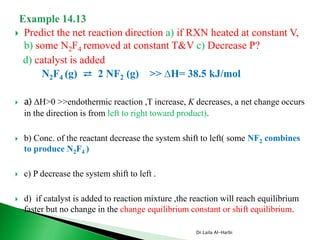1 de 26

### Chapter 14

• 1.  14.1 the concept of equilibrium and the equilibrium constant  14.4 writing equilibrium constant expression  14.4 what does the equilibrium constant tell us?  14.5 factors that effect chemical equilibrium Dr.Laila Al-Harbi
• 2. Equilibrium is a state in which there are no observable changes as time goes by. Chemical equilibrium is achieved when: • the rates of the forward and reverse reactions are equal and the concentrations of the reactants and products remain constant Physical equilibrium H2O (l) Chemical equilibrium N2O4 (g)H2O (g) 2NO2 (g) Dr.Laila Al-Harbi
• 3. • [A], [B], etc. are the equilibrium concentrations • K ≈ [products] / [reactants] • K >> 1 ; favors products >>> Lie to the right • K << 1 favors reactants >>> Lie to the left • K ≈ 1: roughly equal concentration of reactants and products • K is a constant at a given temperature • Solids drop out of the expression & water drops out when the solvent is water • K has no unit K = [C]c [D]d [A]a [B]b conc. of products conc. of reactants equilibrium constant Dr.Laila Al-Harbi
• 4.  A. Homogeneous Equilibria (all species are in the same phase) N2O4 (g) 2NO2 (g) Kc = [NO2]2 [N2O4] Kp = NO2 P 2 N2O4 P aA (g) + bB (g) cC (g) + dD (g) Kp = Kc(RT) ∆n In most cases Kc  Kp ∆n = moles of gaseous products – moles of gaseous reactants ∆n = (c + d) products– (a + b)reactants Kp = Kc , when ∆n= 0 Dr.Laila Al-Harbi
• 5.  Homogeneous Equilibria (all species are in the same phase) CH3COOH (aq) + H2O (l) CH3COO- (aq) + H3O+ (aq) Kc =‘ [CH3COO-][H3O+] [CH3COOH][H2O] [H2O] = constant Kc = [CH3COO-][H3O+] [CH3COOH] = Kc [H2O]‘ General practice not to include units for the equilibrium constant. Dr.Laila Al-Harbi
• 6. The concentration of solids and pure liquids are not included in the expression for the equilibrium constant. CaCO3 (s) CaO (s) + CO2 (g) Kc =‘ [CaO][CO2] [CaCO3] [CaCO3] = constant [CaO] = constant Kc = [CO2] = Kc x‘ [CaCO3] [CaO] Kp = PCO 2 Dr.Laila Al-Harbi
• 7.  Write the equilibrium constant expression for the following reactions: (a) HF (aq) + H2O (ℓ) ⇄ H3O+ (aq) + F- (aq) (b) 2 NO (g) + O2 (g) ⇄ 2 NO2 (g) (c) CH3COOH (aq) + C2H5COH (aq) ⇄ CH3COOC2H5+ H2O (ℓ) Kc = [H3O+ ][F-] [HF] Kp = P 2 NO2 P 2 NO P 2 O2 Kc = [CH3COOC2H5] [CH3COOH] [C2H5COH] Kc = [NO2]2 [NO]2[O2] Homogeneous Dr.Laila Al-Harbi
• 8.  The equilibrium concentrations 2 NO (g) + O2 (g) ⇄ 2 NO2 (g) at 2300C are [NO] = 0.0542 M , [O2] = 0.127 M, and [NO2] = 15.5 M. Calculate the equilibrium constant Kc. Kc = [NO2]2 [NO]2[O2] Kc = [15.5]2 [0.0542]2[0.127] = 6.44 × 105 Homogeneous Dr.Laila Al-Harbi
• 9.  The equilibrium constant Kp for the reaction  is 158 at 1000K. What is the equilibrium pressure of O2 if the PNO = 0.400 atm and PNO = 0.270 atm? 2NO2 (g) ⇄ 2NO (g) + O2 (g) Kp = 2 PNO PO 2 PNO 2 2 PO2 = Kp PNO 2 2 PNO 2 PO2 = 158 x (0.400)2/(0.270)2 = 347 atm Homogeneous Dr.Laila Al-Harbi
• 10.  Methanol is manufactured industrially by the reaction Kc = 10.5 at 220°C. What is the value of Kp at this temperature Kp = Kc (RT)∆n ∆n = 1-3 = -2 Kp = Kc (0.0821× 493)-2 = 6.41 × 10-3 CO (g) + 2 H2 (g) ⇄ CH3OH(g) Homogeneous Dr.Laila Al-Harbi
• 11.  Write the equilibrium constant expression for the following reactions: (a) (NH4)2Se (s) ⇄ 2NH3 (g) + H2Se (g) (b) AgCl (s) ⇄ Ag+ (aq) + Cl- (aq) (c) P4 (s) + 6Cl2(g) ⇄ 4PCl3(ℓ) Kc = [Ag+ ]2 [Cl-] Kp = PNH3 2 PH2SeKc = [NH3]2 [H2Se] Kc = 1 [Cl2]6 Heterogeneous Dr.Laila Al-Harbi
• 12. Consider the following equilibrium at 295 K: The partial pressure of each gas is 0.265 atm. Calculate Kp and Kc for the reaction? NH4HS (s) ⇄ NH3 (g) + H2S (g) Kp = P NH3H2S P = 0.265 x 0.265 = 0.0702 Kp = Kc(RT)Dn Kc = Kp(RT)-Dn ∆n = 2 – 0 = 2 T = 295 K Kc = 0.0702 x (0.0821 x 295)-2 = 1.20 x 10-4 Example 14-6 Heterogeneous Dr.Laila Al-Harbi
• 13.  A) Predicting the direction of a reaction  The reaction quotient (Qc) is calculated by substituting the initial concentrations of the reactants and products into the equilibrium constant (Kc) expression.  IF • Qc > Kc system proceeds from right to left to reach equilibrium • Qc = Kc the system is at equilibrium • Qc < Kc system proceeds from left to right to reach equilibrium  B) Calculating equilibrium concentration 1. Express the equilibrium concentrations of all species in terms of the initial concentrations and a single unknown x, which represents the change in concentration. 2. Write the equilibrium constant expression in terms of the equilibrium concentrations. Knowing the value of the equilibrium constant, solve for x. 3. Having solved for x, calculate the equilibrium concentrations of all species. Dr.Laila Al-Harbi
• 14. Q = = 0.35 0.25 = 1.40 To reach equilibrium [iso-Butane] must increase and [n-Butane] must decrease. Since Q (1.4) < Kc (2.5) , the system at equilibrium [iso] [n] Q has the same form as K, . . . but uses existing concentrations n-Butane iso-Butane 0.25 0.35 Kc =2.5 Q = A) Predicting the direction of a reaction Dr.Laila Al-Harbi
• 15.  0.249mol,N2 , 3.2×10-2 mol H2 , 6.42×10-4 mol NH3in A 3.50 L at 375°C , kc= 1.2, Decide whether the system is at equilibrium .if it is not predict which way the net reaction will proceed. N2 (g) + 3H2(g) ⇄ 2NH3 (g) Homogeneous [NH3]2 [N2] [H2]3 Q c= Since the molarity = number of moles /volume in L , M=n/Vin L [N2] °= 0.249/3.50 L = 0.0711 M [H2] ° = 3.2×10-2 /3.50 L = 9.17 ×10-3 M [NH3] ° = 6.42×10-4 /3.50 L = 1.83 ×10-4 M = [1.83 ×10-4 ]2 [0.0711][9.17 ×10-3]2 = 0.611Q c= [NH3]2 [N2] [H2]3 Since Q (0.611) < Kc (1.2) system , the system at equilibrium To reach equilibrium [NH3] must increase and [N2], [H2] must decrease. The net reaction will proceed from left to right untial equilibrium is reached . Dr.Laila Al-Harbi
• 16. cis-stilbene trans-stilbene Kc =24 B) Calculating equilibrium concentration Step 2 Put equilibrium Conic. into Kc . Step 1 Define equilibrium condition in terms of initial condition and a change variable [cis-stibene] [trans-stibene] Initial 0.85 0 At equilibrium 0.85-x x Step 3. Solve for x. 24(0.85-x) = x = 20.4 – 24x=x 25x= 20.4 >>>>>> x = 0.816 M [cis-stilbene] = 0.85 – 0.816 = 0.034 M [trans-stilbene] = x = 0.816 M At equilibrium Dr.Laila Al-Harbi
• 17. Step 2 Put equilibrium Conic. into Kc . Step 1 Define equilibrium condition in terms of initial condition and a change variable [H2] [I2] [HI] Initial x x 0 At equilibrium 0.5-x 0.5-x 2x Step 3. Solve for x. 54.3 = (2x)2/(0.5-x)2 Square root of both sides & solve gives: 7.369 = 2x/0.5-x x = 0.393 [H2] = [I2] = 0.5 – 0.393 = 0.107 M [HI] = 2x = 0.786 M At equilibrium M= 0.5/1 =0.5 mol Dr.Laila Al-Harbi
• 18.  Consider the reaction in Ex.14.9 starting with [HI] =0.04 molar . Calculate [HI],[I2]& [H2] at equilibrium. 2 HI(g) ⇄ H2(g) + I2(g) Kc = 1/ 54.3 = 0.0184 [HI] [I2] [H2] Initial 0.04 0 0 At equilibrium 0.04-2x x x [I2][H2] [HI]2Kc = x2 (0.04-2x)2 = = 0.0184 Square root of both sides & solve gives: x = 0.0043 M At equilibrium [H2] = [I2] = 0.0043 = 0.0043 M [HI] = 0.04- 2x = 0.0031 M Dr.Laila Al-Harbi
• 19.  Le Chatelier’s principle , If an external stress is applied to a system at equilibrium, the system adjusts in such a way that the stress is partially offset as the system reaches a new equilibrium position. factors that effect chemical equilibrium 1. Changes in Concentration 2. Changes in Volume and Pressure 3. Changes in Temperature 4. Adding a Catalyst Dr.Laila Al-Harbi
• 20. N2 (g) + 3H2 (g) 2NH3 (g) Add NH3 Equilibrium shifts left to offset stress Change Shifts the Equilibrium Increase concentration of product(s) left Decrease concentration of product(s) right Decrease concentration of reactant(s) Increase concentration of reactant(s) right left Dr.Laila Al-Harbi
• 21.  N2 (g) + 3H2(g) ⇄ 2NH3 (g) >>>> Kc=2.37×10-3 , T=720°C [N2] = 0.683 M [H2] = 8.800M [NH3] = 1.05M the concentration increase to 3.65 M Add NH3 Equilibrium shifts left to offset stress 3.65 M Calculate Qc compare it value with Kc because Qc (2.86×10-2) > Kc (2.37×10-3) , The net reaction direction from right to left until Qc = Kc Dr.Laila Al-Harbi
• 22. A (g) + B (g) C (g) Change Shifts the Equilibrium Increase pressure (decrease volume) Side with fewest moles of gas Decrease pressure (Increase volume) Side with most moles of gas ∆n=n products –n reactants Note: Pressure and volume are inversely proportional. ∆n = is the number of moles for substance in gaseous products &gaseous reactants Because the pressure of gases is related directly to the concentration by P = n/V, changing the pressure by increasing/decreasing the volume of a container will disturb an equilibrium system. Dr.Laila Al-Harbi
• 23.  Predict the net reaction direction (increasing P & decreasing V) (a) 2 PbS (s) + 3O2 (g) ⇄ 2PbO (s) + 2HS2 (g) ∆n=n products –n reactants = 2-3 = -1 When the volume of an equilibrium mixture of gases is reduced, a net change occurs in the direction that produces fewer moles of gas (left to right toward product). (b) PCl5 (g) ⇄ PCl3(g) + Cl2 (g) ∆n=n products –n reactants = 2-1 =1 When the volume of an equilibrium mixture of gases is reduced, a net change occurs in the direction that produces fewer moles of gas (right to left toward reactant). (c) H2 (g) + CO2(g) ⇄ H2O(g) + CO(g) ∆n=n products –n reactants = 2-2 = 0 The change in P,v has no effect on the equilibrium . Dr.Laila Al-Harbi
• 24. Change Exothermic Rx Increase temperature K decreases Decrease temperature K increases Endothermic Rx K increases K decreases •Adding a Catalyst • does not change K • does not shift the position of an equilibrium system • system will reach equilibrium sooner uncatalyzed catalyzed Catalyst lowers Ea for both forward and reverse reactions Catalyst does not change equilibrium constant or shift equilibrium. Dr.Laila Al-Harbi
• 25. Le Châtelier’s Principle Change Shift Equilibrium Change Equilibrium Constant Concentration yes no Pressure yes no Volume yes no Temperature yes yes Catalyst no no Dr.Laila Al-Harbi
• 26.  Predict the net reaction direction a) if RXN heated at constant V, b) some N2F4 removed at constant T&V c) Decrease P? d) catalyst is added N2F4 (g) ⇄ 2 NF2 (g) >> ∆H= 38.5 kJ/mol  a) ∆H>0 >>endothermic reaction ,T increase, K decreases, a net change occurs in the direction is from left to right toward product).  b) Conc. of the reactant decrease the system shift to left( some NF2 combines to produce N2F4 )  c) P decrease the system shift to left .  d) if catalyst is added to reaction mixture ,the reaction will reach equilibrium faster but no change in the change equilibrium constant or shift equilibrium. Dr.Laila Al-Harbi